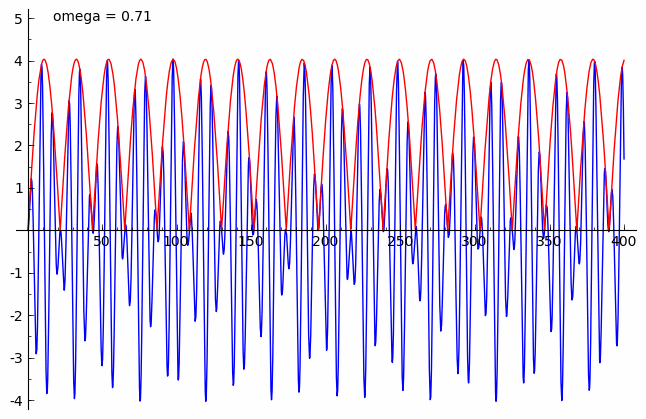jsMath

# Forced vibrations

 ```This example illustrates the relationship between the solution of a differential equation modeling forced vibration and the forcing function. Here we consider the initial value problem y′′+0.125y′+y= 3cos(ωt),y(0)=0,y′(0)=2. In blue is the solution to the IVP, while in red is the forcing function F(t)=3cos(ωt). Here you can see how different values of ω affect the amplitude of the solution. Here are plots for various values of ω: ω=0.3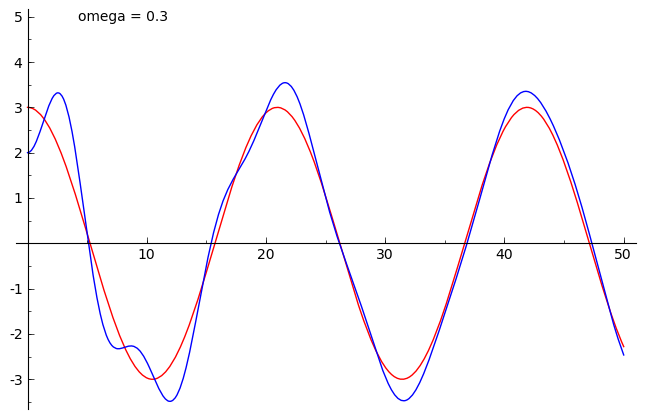ω=1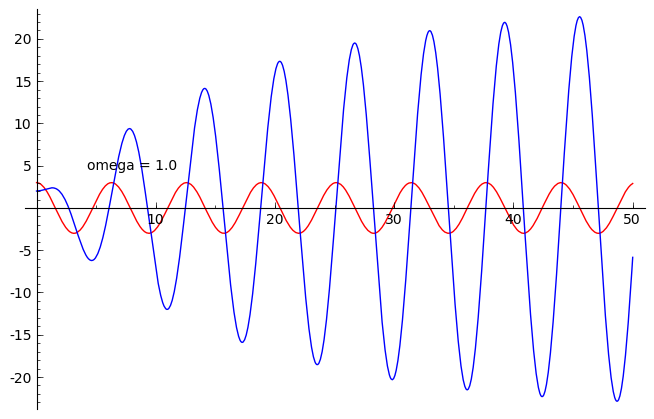ω=3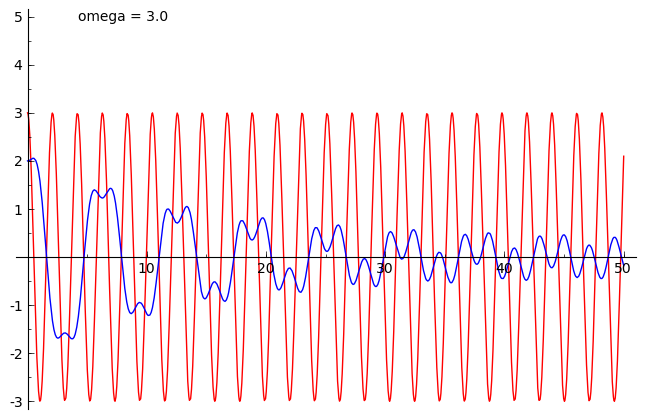Here is an animation showing the solution for many values of ω:```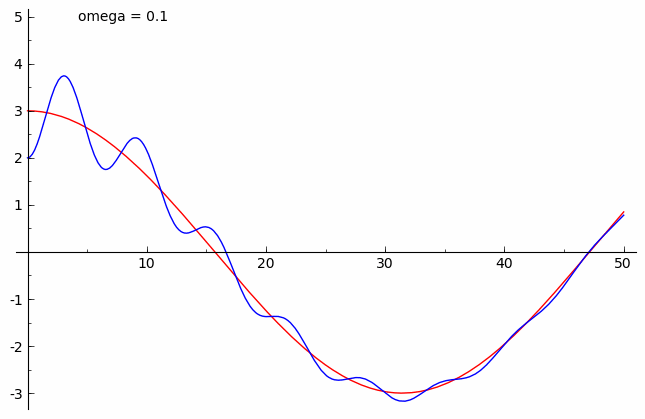```This example illustrates periodic variation of amplitude which occurs when you have a forced, undamped vibration. Suppose a vibration is modelled by y′′+y′=cos(ωt),y(0)=0,y′(0)=0. Then the solution will be y=(21−ω2sin(2(1−ω)t))sin(2(1+ω)t).  If ω is close to 1, then 1−ω  is small, and hence the factor sin(2(1+ω)t)  is oscillating much faster than 21−ω2sin(2(1−ω)t).  So, we think of | |  21−ω2sin(2(1−ω)t)| |    (shown in red) as periodically varying the amplitude of the sine curve. Here are plots for various values of ω: ω=0.7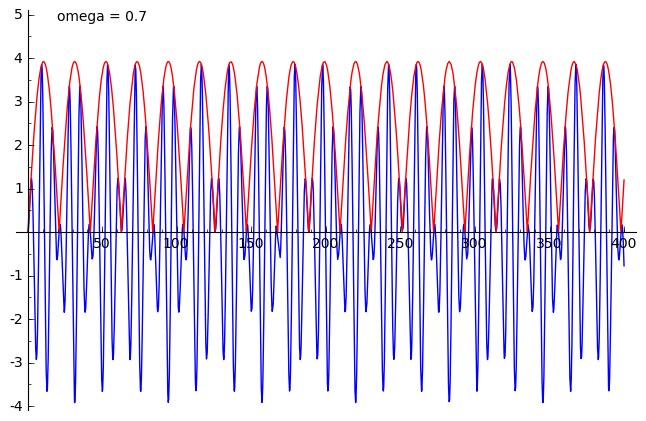ω=0.9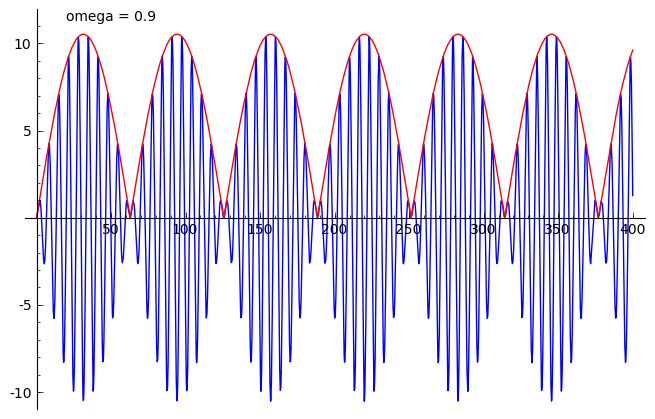ω=0.95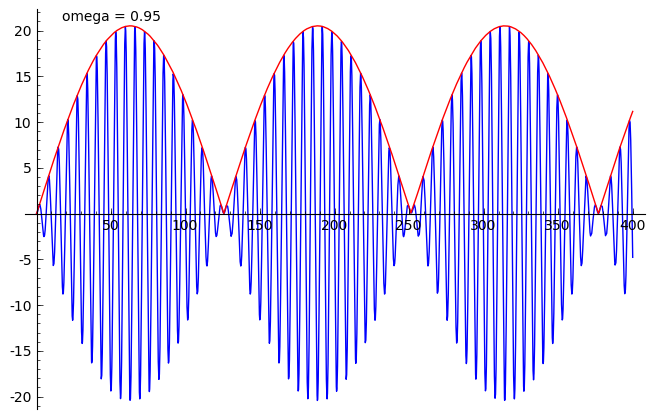Here's an animation showing the solution for many values of ω:```